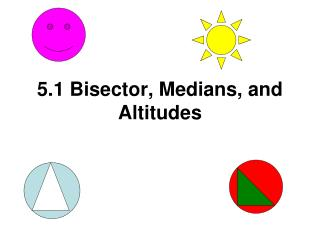Download Presentation5.1 Bisector, Medians, and Altitudes# 5.1 Bisector, Medians, and Altitudes - PowerPoint PPT Presentation

Download Presentation##### 5.1 Bisector, Medians, and Altitudes

Download Policy: Content on the Website is provided to you AS IS for your information and personal use and may not be sold / licensed / shared on other websites without getting consent from its author. While downloading, if for some reason you are not able to download a presentation, the publisher may have deleted the file from their server.

- - - - - - - - - - - - - - - - - - - - - - - - - - - E N D - - - - - - - - - - - - - - - - - - - - - - - - - - -
##### Presentation Transcript

1. 5.1 Bisector, Medians, and Altitudes

2. A 3x + 16 6x - 5 B C D B. Theorem 5.1: If a point lies on the perpendicular bisector of a segment, then the point is equidistant from the endpoints of the segment. Example 1: Find x, if AD is a perpendicular bisector.

3. B E C A C. Theorem 5.3: Circumcenter TheoremThe circumcenter of a triangle is equidistant from the vertices of the triangle. Example 2 Find x and y, if BE = 24, AE = 3x – 6 and CE = 5y + 4

4. D. Theorem 5.4: If a point lies on the bisector of an angle, then the point is equidistant from the sides of the angle. Example 3 Find x, if AC is an angle bisector of  DAB and DC = x + 4 and BC = 2x – 5. D C A B

5. E T S A D U F E. Theorem 5.7: Centroid TheoremThe distance from the vertex to the point of concurrency is equal to 2 times the distance from the point of concurrency to the midpoint of the opposite side. Example 4 Point A is a centroid of ∆DEF. Find x, y, and z. SA = 4z EA = y TA = 2x – 5 FA = 4.6 UA = 2.9 DA = 6